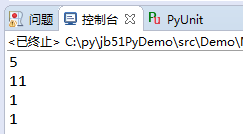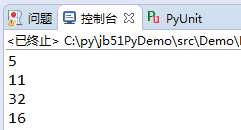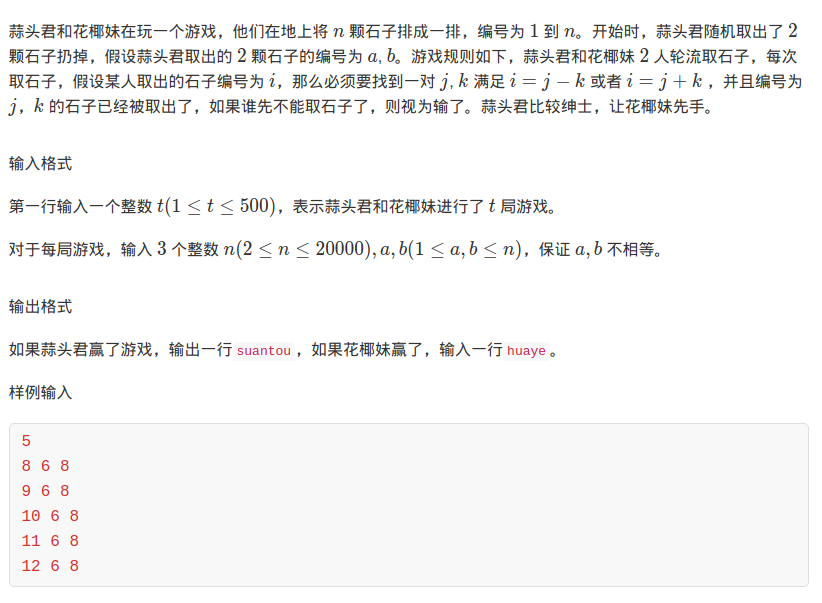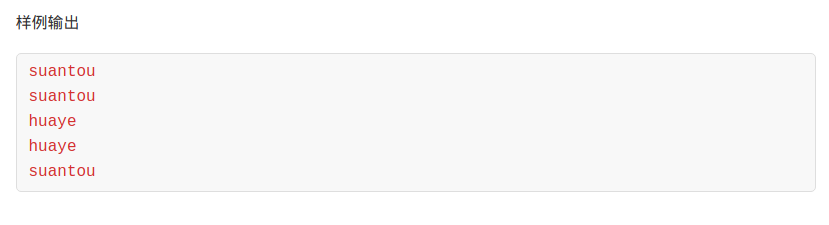• 主要介绍了Python基于更相减损术实现求解最大公约数的方法,简单说明了更相减损术的概念、原理并结合Python实例形式分析了基于更相减损术实现求解最大公约数的相关操作技巧与注意事项,需要的朋友可以参考下
• 本文实例讲述了Python基于更相减损术实现求解最大公约数的方法。分享给大家供大家参考，具体如下：先从网上摘录一段算法的描述如下：更相减损法：也叫 更相减损术，是出自《 九章算术》的一种求最大公约数的算法，它...
本文实例讲述了Python基于更相减损术实现求解最大公约数的方法。分享给大家供大家参考，具体如下：先从网上摘录一段算法的描述如下：更相减损法：也叫 更相减损术，是出自《 九章算术》的一种求最大公约数的算法，它原本是为 约分而设计的，但它适用于任何需要求最大公约数的场合。《九章算术》是中国古代的数学专著，其中的“更相减损术”可以用来求两个数的最大公约数，即“可半者半之，不可半者，副置分母、子之数，以少减多，更相减损，求其等也。以等数约之。”翻译成现代语言如下：第一步：任意给定两个正整数；判断它们是否都是偶数。若是，则用2约简；若不是则执行第二步。第二步：以较大的数减较小的数，接着把所得的差与较小的数比较，并以大数减小数。继续这个操作，直到所得的减数和差相等为止。看完上面的描述，我的第一反应是这个描述是不是有问题？从普适性来说的话，应该是有问题的。举例来说，如果我求解4和4的最大公约数，可半者半之之后，结果肯定错了！后面的算法也不能够进行！不管怎么说，先实现一下上面的算法描述：# -*- coding:utf-8 -*-#! python2def MaxCommDivisor(m,n):# even processwhile m % 2 == 0 and n % 2 == 0:m = m / 2n = n / 2# exchange order when neededif m < n:m,n = n,m# calculate the max comm divisorwhile m - n != n:diff = m - nif diff > n:m = diffelse:m = nn = diffreturn nprint(MaxCommDivisor(55,120))print(MaxCommDivisor(55,77))print(MaxCommDivisor(32,64))print(MaxCommDivisor(16,128))运行结果：不用说，上面程序执行错误百出。那么该如何更正呢？首先，除的2最终都应该再算回去！这样，程序修改如下：def MaxCommDivisor(m,n):com_factor = 1if m == n:return nelse:# process for even numberwhile m % 2 == 0 and n % 2 == 0:m = int(m / 2)n = int(n / 2)com_factor *= 2if m < n:m,n = n,mdiff = m - nwhile n != diff:m = diffif m < n:m,n = n,mdiff = m - nreturn n * com_factorprint(MaxCommDivisor(55,120))print(MaxCommDivisor(55,77))print(MaxCommDivisor(32,64))print(MaxCommDivisor(16,128))通过修改，上面程序执行结果如下虽说这段程序写出来看着有点怪怪的，但是总体的算法还是实现了。与辗转相除等算法相比，这个在循环的层级上有一定的概率会减小。特别是最后的两组测试数字对儿，这种情况下的效果要好一些。但是，总体上的算法的效率，现在我还不能够给个准确的衡量。PS：这里再为大家推荐几款计算工具供大家进一步参考借鉴：希望本文所述对大家Python程序设计有所帮助。
展开全文• 利用更相减损术求两个大数的最大公约数 这个题我没有过。。BZOJ1876 原因有这么几个： 如果使用十进制高精度配合普通更相减损术之后会T4个点 如果使用十进制高精度配合优化之后的更相减损术会T7个点 如果使用万...
利用更相减损术求两个大数的最大公约数
这个题我没有过。。BZOJ1876
原因有这么几个：
如果使用十进制高精度配合普通更相减损术之后会T4个点
如果使用十进制高精度配合优化之后的更相减损术会T7个点
如果使用万进制高精度配合普通更相减损术会全T
最后一种不用再试了
原因我分析了一下，封装之后的十进制高精度对优化的支持不是特别好，可能要写底层操作
万进制高精度的板子玩不来
所以这道题，只给出更相减损术的普通写法和优化写法，代码不放了
等以后万进制高精度学会了再复刷此题

bign gcd1(bign m,bign n)
{
if(m==n) return m;
if(m>n) return gcd1(n,m-n);
return gcd1(m,n-m);
}

这是最简单的递归形式的，但是有个缺点，不断的传参复制复制复制，然后就会MLE的
而且递归也没办法传引用
所以立刻改成了非递归形式

bign gcd2(bign &m,bign &n)
{
bign t;
while(!(m==n))
{
if(m>n)
{
t=m;
m=n;
n=t-n;
}
else
{
n=n-m;
}
}
return m;
}

改的还是很合理的，效率也不错
然后咱们学习一下优化算法：

如果两个数都是偶数，那么他们一定有2这个质因子，提取2直到两个数有一个是奇数
一奇一偶的话，提取那个偶数的2直到它变成奇数，因为奇数和它的公因子不可能是2
然后就是两个奇数的情况，用一个减另一个，就变成了一奇一偶的情况，然后处理方法同上
最后是剩下一个数和0，将剩下的这个数乘上一开始两个数一起提取的2，就是答案

实现的话，我找了一分，可能找的这份效率不是特别好，但是正确性有保证，其实现思路和算法描述的一致：

bign gcd(bign &x,bign &y)
{
int g=0;
bign zero=0;
bool x1,y1;
while(!(x==zero)&&!(y==zero))
{
x1=!((x.s)&1),y1=!((y.s)&1);
if(x1&&y1) {g++;x=x/2;y=y/2;}
else if(x1||y1)
{
if(x1) x=x/2;
else y=y/2;
}
else if (y<x) x=x-y;
else y=y-x;
}
if(x<y) x=y;
while(g--) x=x*2;
return x;
}

不过还是跪了，这个题确实需要返工了
转载于:https://www.cnblogs.com/aininot260/p/9479732.html
展开全文• 求解两个int类型数字a, b的最大公约数的常用的办法一般有两种：第一种是辗转相除术，第二种是更相减损术，下面使用更相减损术求解最大公约数： 设A > B，A = ax, B = bx 则C = A - B = ax - bx，所以A、B、C的...
求解两个int类型数字a, b的最大公约数的常用的办法一般有两种：第一种是辗转相除术，第二种是更相减损术，下面使用更相减损术求解最大公约数：

设A > B，A = ax, B = bx 则C = A - B = ax - bx，所以A、B、C的最大公约数也为x，所以可以转换为：

如果A > B，则 gcd(A,B) = gcd(B,A-B)
如果A < B，则 gcd(A,B) = gcd(A,B-A)

下面是具体的程序代码：

class Solution:
def gcd(self, a: int, b: int) -> int:
while a != b:
if a > b:
a = a - b
else:
b = b - a
return a

if __name__ == '__main__':
print(Solution().gcd(eval(input()), eval(input())))


展开全文• #include &lt;iostream&gt; #include &lt;cstdio&gt; #include&.../*algorithm内置了最大公约数模板__gcd(a,b.../*提示说是欧几里得，坑我看了老半天，结果发现是更相减损术*/ int main() { int t...#include <iostream>
#include <cstdio>
#include<algorithm>
using namespace std;
/*algorithm内置了最大公约数模板__gcd(a,b),注意gcd前面有两道下划线。*/
/*提示说是欧几里得，坑我看了老半天，结果发现是更相减损术*/
int main()
{
int t;
scanf("%d",&t);
while(t--){
int n,a,b;
scanf("%d%d%d",&n,&a,&b);
int x=__gcd(a,b);
int sum=0;
for(int i=1;i<=n;i++){
if(i!=a&&i!=b){
if(i%x==0) sum++;
}
}
if(sum%2) printf("huaye\n");
else printf("suantou\n");
}
return 0;
}

展开全文• public class 最大公约数_更相减损术 { /** * @param args */ public static int getGreatestCommonDivisorV3(int a, int b) { if (a == b) { return a; } int big = a > b ? a : b; int small...java 算法
• 最大比例 题意 给出一个等比数列中的几项，求出符合这几项的最大等比值。...转化为：求这个新数列的最大公约数，因为有指数的形式，采用 更相减损术求这些指数的最大公约数。 代码 在这里插入代码片 ...
• 高中学过的求大公约数的方法就是辗转相除法和更相减损术了。 辗转相除法递归版 #include <iostream> using std::cin; using std::cout; using std::endl; int gcd(int a, int b) { return b == 0 ? a : gcd(b...算法
• 更相减损术 更相减损术是出自《九章算术》的一种求最大公约数的算法，它原本是为约分而设计的，但它适用于任何需要求最大公约数的场合。 出处 《九章算术》 用途 求最大公约数 作用 适用任何需要求最大公约数...
• 更相减损术出自我国著名算数书《九章算术》，原本是用于分数的约分，后多用来计算两个整数的最大公约数。 具体步骤： 第一步：给定两个正整数，判断是否为偶数，若是则用2简约，否则执行第二步。 第二步：以较大的数...最大公约数
• 根据更相减损术，若 gcd(N,x)=1gcd(N,x)=1gcd(N,x)=1，则有 gcd(N,N−x)gcd(N,N-x)gcd(N,N−x)=1，那么考虑成对的与 NNN 互素的数，则答案为 (N−1)×N/2−euler(N)∗N/2(N-1)\times N/2 - euler(N)*N/2(N−1)×N/2...
• 本文描述 乘法逆元、更相减损术、辗转相除法和拓展欧几里得算法。 更相减损术与辗转相除法 两个算法多用于计算最大公约数，经由拓展方式处理可以用于计算乘法逆元。以下为算法描述： 更相减损术 记数字 A=8，数字 B=...算法 数学问题 乘法逆元
• 更相减损法：也叫 更相减损术，是出自《 九章算术》的一种求最大公约数的算法，它原本是为 约分而设计的，但它适用于任何需要求最大公约数的场合。 《九章算术》是中国古代的数学专著，其中的“更相减损术”可以...
• 题意 传送门 NC 26255 题解 只考虑后两种操作很容易用线段树维护，...根据更相减损术（类似于减法版的辗转相除法），最大公约数有如下性质 gcd(x,y,z… )=gcd(x,y−x,z−y,… )gcd(x,y,z\dots)=gcd(x,y-x,z-y,\dots)g
• 点击我观看“百度百科-更相减损术”，对于更相减损术，百度百科肯定解释的比我好，当然我也看了百度的解释，大家慢慢食用，理解之后在来看以下代码实现就可以了！ 编码实现： public class Test { public static ...
•c语言
• 思路:a+b a-b所得出的为等差数列，其中等差数列的第一项 即为gcd(a,b) 因为该相减过程和 更相减损术类似 所以直接求出最大公约数 然后用N/gcd(a,b) - 2如果为奇数则先走的赢 如果为偶数则后走的赢 ...
• * 使用更相减损术求最大公约数 * 第一步：任意给定两个正整数；判断它们是否都是偶数。若是，则用2约简；若不是则执行第二步。 * 第二步：以较大的数减较小的数，接着把所得的差与较小的数比较，并以大数减小数。...最大公约数
• 《九章算术》中介绍了这个方法,叫做”更相减损术”,即“可半者半之，不可半者，副置分母、子之数，以少减多，更相减损，求其等也。以等数约之。” 　翻译成现代语言如下： 　第一步：任意给定两个正整数；判断它们...
• 求两个数的最大公约数的方法：辗转相除法和更相减损术
• 辗转相除法：两个正整数a和b（a&gt;b），它们的最大公约数等于a除以b的余数c...更相减损术：两个正整数a和b（a&gt;b），它们的最大公约数等于a-b的差值c和较小数b的最大公约数。比如10和25，25减去10的差是...辗转相除法 求最大公约数 数据结构与算法...